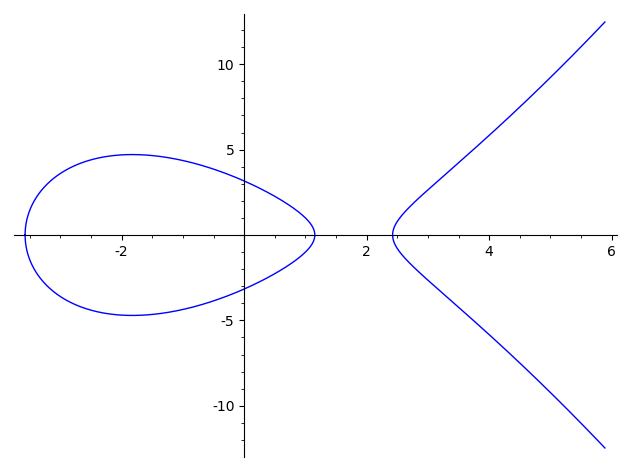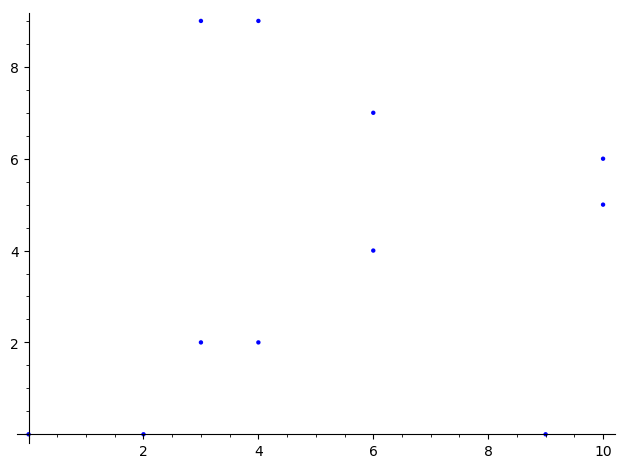# Using elliptic curves and isogenies in Sage¶

## Finite fields¶

We create finite fields by passing their cardinality

In :
Fp = GF(11)

In :
Fp

Out:
Finite Field of size 11
In :
Fq = GF(11^2)
Fq

Out:
Finite Field in z2 of size 11^2

For extension fields, the generator is obtained with the .gen() function.

In :
z = Fq.gen()
z

Out:
z2
In :
z^120

Out:
1

Same thing in one go

In :
K.<t> = GF(next_prime(2^128)^2)
K

Out:
Finite Field in t of size 340282366920938463463374607431768211507^2

## Elliptic curves¶

Curves over $ℚ$

In :
E = EllipticCurve([-10,10])
E

Out:
Elliptic Curve defined by y^2 = x^3 - 10*x + 10 over Rational Field
In :
E.plot()

Out:Cuvers over other fields

In :
F = EllipticCurve(GF(11), [1, 0])
F

Out:
Elliptic Curve defined by y^2 = x^3 + x over Finite Field of size 11
In :
F.order()

Out:
12
In :
F.cardinality()

Out:
12
In :
F.points()

Out:
[(0 : 0 : 1), (0 : 1 : 0), (5 : 3 : 1), (5 : 8 : 1), (7 : 3 : 1), (7 : 8 : 1), (8 : 5 : 1), (8 : 6 : 1), (9 : 1 : 1), (9 : 10 : 1), (10 : 3 : 1), (10 : 8 : 1)]
In :
P = F.random_point()
P

Out:
(9 : 1 : 1)
In :
P.order()

Out:
6

Group structure

In :
F.abelian_group()

Out:
Additive abelian group isomorphic to Z/12 embedded in Abelian group of points on Elliptic Curve defined by y^2 = x^3 + x over Finite Field of size 11
In :
g = F.gens()
g

Out:
(8 : 6 : 1)
In :
g.order()

Out:
12

Construct an isogeny with given kernel

In :
origin = 6*g
origin

Out:
(0 : 0 : 1)
In :
F.point([0,0])

Out:
(0 : 0 : 1)
In :
I = F.isogeny(origin)
I

Out:
Isogeny of degree 2 from Elliptic Curve defined by y^2 = x^3 + x over Finite Field of size 11 to Elliptic Curve defined by y^2 = x^3 + 7*x over Finite Field of size 11
In :
I.rational_maps()

Out:
((x^2 + 1)/x, (x^2*y - y)/x^2)
In :
FF = I.codomain()

In :
FF

Out:
Elliptic Curve defined by y^2 = x^3 + 7*x over Finite Field of size 11
In :
FF.abelian_group()

Out:
Additive abelian group isomorphic to Z/6 + Z/2 embedded in Abelian group of points on Elliptic Curve defined by y^2 = x^3 + 7*x over Finite Field of size 11
In :
FF.plot()

Out:The same example, over the rationals

In :
E = EllipticCurve([1,0])

In :
P = E.lift_x(0)
P

Out:
(0 : 0 : 1)
In :
P.order()

Out:
2
In :
J = E.isogeny(P)
EE = J.codomain()
EE

Out:
Elliptic Curve defined by y^2 = x^3 - 4*x over Rational Field

In (very) limited cases, Sage can compute the isogeny given the image curve and the degree

In :
JJ = E.isogeny(None, codomain=EE, degree=2)

In :
J == JJ

Out:
True

## Your turn: exercices!¶

### Diffie-Hellman¶

Implement the (naive) Diffie-Helman key exchange scheme with elliptic curves.

#### 1. Find a curve of prime order¶

Choose a prime $p$. Take curves at random over $𝔽_p$ until you find one with prime order.

Suggestion: For a start, take $p$ of ~60 bits. When your code works well enough you can try 160 bits (it may take a few minutes to find a curve)

In [ ]:



#### 2. Implement the scheme¶

Write a function to sample secret keys, and a function to produce public keys. Perform the key exchnage and verify that Alice and Bob obtain the same key.

In [ ]:



### ECM Factoring method¶

Implement Lenstra's factoring method. See the lecture notes, Section 5.

Note: Sage has limited support for curves over $ℤ/Nℤ$, just enough to implement ECM.

In [ ]:



### Generating irreducible polynomials¶

Implement the Couveignes-Lercier method for generating irreducible polynomials. See the lecture notes, Section 8.

Note: Sage has limited support for curves over $ℤ/Nℤ$, just enough to implement ECM.

In [ ]: